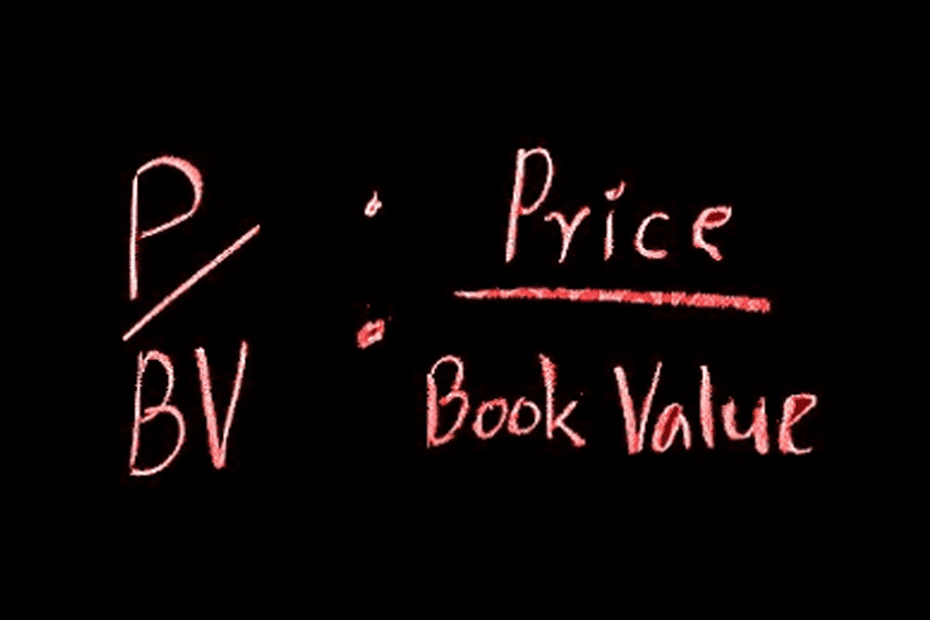Home » Blog » Investing » Stock Market Basics » Price To Book Value Ratio Significance For Investors

# Price To Book Value Ratio Significance For Investors

• Stock Market BasicsIn our last post, we have understood what Book Value is. Now once again, price to book value ratio significance is an entirely vital concept for evaluating stock price.  In order to understand the P/BV ratio, we need to convey some brief information about book value and market value separately. Price to book value ratio refers ratio of current market value and book value. It guides investors to evaluate a company’s market value relative to its book value.

Market value is the current worth of stocks while BV interprets the actual worth. Book value reflects a stock’s fair value but market value indicates the current estimated value based on the current trend and the quotes received from the stock exchange. Market value is generally higher than book value but one can not pre-decide it.

Therefore, we calculate the price to BV ratio by dividing the current market value by the last quarter’s book value per share. This is basically a comparison to evaluate the undervalued and overvalued stocks. A comparison should be placed between the same sectors. So before calculating the price to book value ratio, we must first learn the concept of book value and market value.

If the price to BV ratio is less than 1.0, the stock is undervalued and if the price to book value ratio is more than 1.0, it is overvalued. Though generally it is advised not to buy overvalued stocks, we should not always decide it based on the price to book ratio. Sometimes investors go for overvalued stocks by evaluating a company’s future. Investors generally avoid underrated stocks but is a company’s future perception is clear and profitable, underrated stocks are also worth buying. Following are some points about the price to book value:

## Beneficial Aspects of Price to Book Value Ratio Significance

• It is useful to calculate the fair value of the stock.
• By comparing the final amount one can predict the current market scenario.
• We generally use this ratio for liquid assets such as investment, finance, banking, insurance, etc.
• It is best to compare the P/BV ratio within the same industry.

### Real Example of Price to Book Value Ratio

This is the current chart of the Infosys share price. The current market value of each share in Infosys Ltd. is Rs. 1171.25 and book value per share is Rs. 315.60. So the price to book value ratio is (1171.25 / 315.60)=  Rs. 3.70. As per this example, Infosys is also currently quoting as overvalued.

So, it is clear that price to book value ratio significance has major importance in the field of investment and especially in fundamental analysis. In the case of market valuation, it plays a significant role.